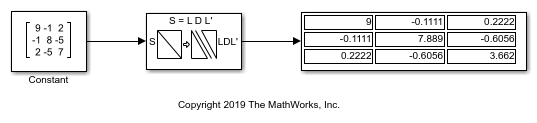# LDL Factorization of 3-by-3 Hermitian Positive Definite Matrix

This example shows how to use LDL Factorization to LDL-factor a 3-by-3 Hermitian positive definite matrix.

For the input in the model, the corresponding L and D values become:

```L = 1.0000 0 0 -0.1100 1.0000 0 0.2200 -0.6100 1.0000 D = 9.0000 0 0 0 7.8900 0 0 0 3.6600 Ltranspose = 1.0000 -0.1100 0.2200 0 1.0000 -0.6100 0 0 1.0000 ```

The output matrix is composed of the bottom left triangle of the `L` matrix, the diagonal of the `D` matrix, and the top right triangle of `Ltranspose`. When they are combined, the output is:

```S = 9.0000 -0.1100 0.2200 -0.1100 7.8900 -0.6100 0.2200 -0.6100 3.6600 ```

In the model, the output is the same.